# Exponents

Exponents, or powers, are a way of indicating that a quantity is to be multiplied by itself some number of times. In the expression 25, the number 2 is the “base” and 5 the exponent, or “power.” For example, “2 to the fifth power” is shorthand for “multiply five 2’s”: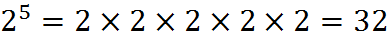The exponent is read “two raised to the fifth power” or “two to the fifth.” Notice that the exponent tells us how many bases to multiply, not how many multiplications to perform (which is one less than the number of bases.)

Exponents are normally written in the form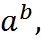where a is the base and b is the exponent. In some contexts, but not on the GMAT, ab is commonly written as “a^b” or less often as “a**b“. When the math gets abstract or confusing, imagine the letters a and b as representing numbers counting the number of multiplications.

## Squared Numbers

A number is to be multiplied by itself (for example, 5 times 5) is squared. To visualize squaring, picture a square which is 5 units long and 5 units wide. This square will then have an area of five squared, or 25, square units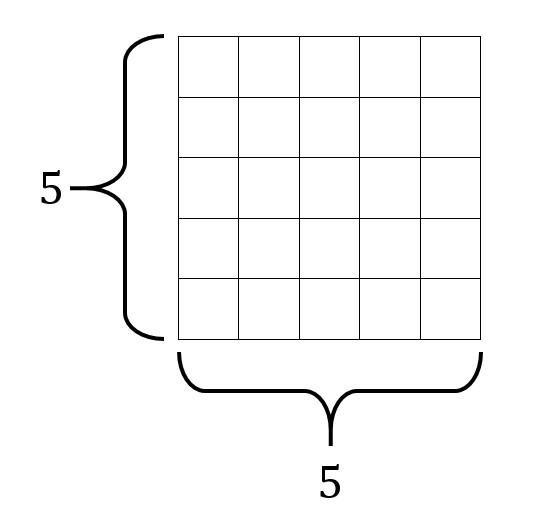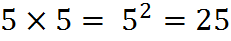For the GMAT, you should memorize these perfect squares: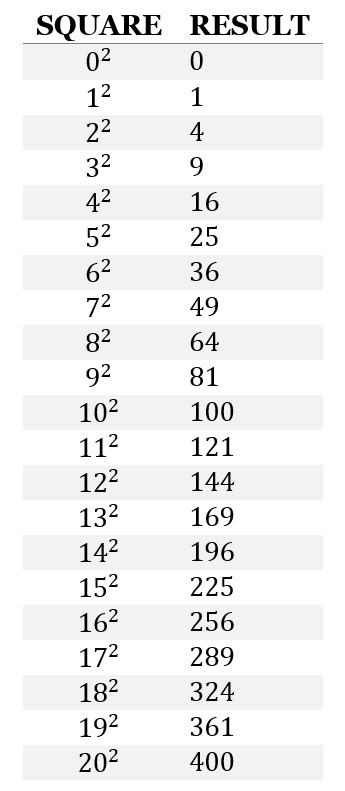## Cubed Numbers

Similarly, a convenient way to note that a number is to be multiplied by itself and then itself again (for example 5 times 5 times 5) is to say that the number is cubed. We write “5 cubed” as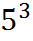where 5 is the base and 3 is the exponent. To help visualize this, picture a cube which is 5 units long, 5 units wide, and 5 units high. This cube will then have a volume of 125 cubic units.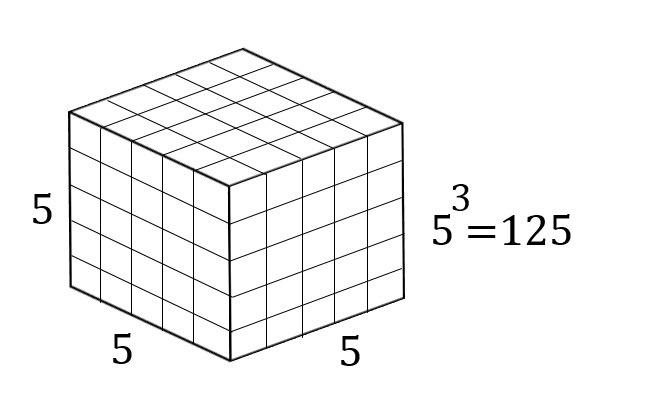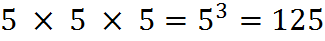For the GMAT, you should memorize the perfect cubes up to at least 5 cubed: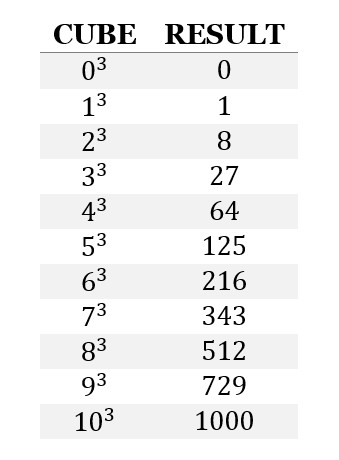## Integer Exponents

When the exponent is a positive integer, then it is just a simple case of multiplying the base by itself a certain number of times. For example,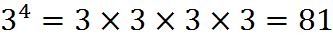Here, 3 is the base, 4 is the exponent (written as a superscript), and 81 is 3 raised to the 4th power. Notice that the base 3 appears 4 times in the repeated multiplication, because the exponent is 4.

Some more examples: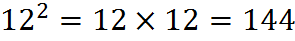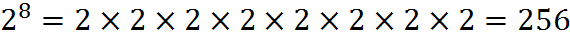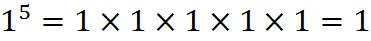## Multiplying and Dividing Exponents

If you have two or more exponents with the same base, then multiplying them has the same effect as adding their exponents. For example,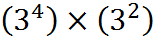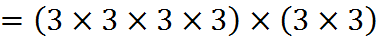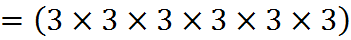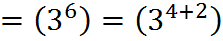Similarly, if you have two or more exponents with the same base, then dividing them has the same effect as subtracting their exponents. For example,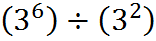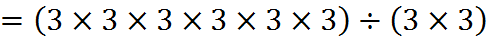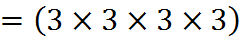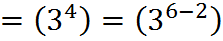## Exercises

1. Compute the following:

a)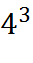b)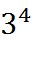c)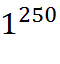d)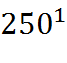2. Write these numbers as powers of 2:

a)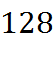b)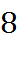c)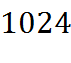3. Evaluate: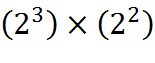4. Evaluate: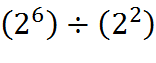5. Why is this true?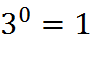(Answers to these exercises are below, in this chapter.)

## Negative and Fractional Exponents

Negative exponents make sense given the division rule. Given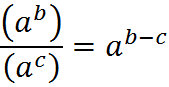if c is greater than b, then we end up with a negative exponent. All the a‘s in the numerator are canceled out, but some remain in the denominator. For example, if we divide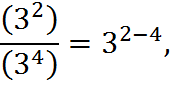we are left with a negative exponent corresponding to two 3’s left in the denominator: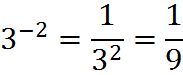Exponents can be fractional as well. With a fractional exponent, the numerator acts as a normal whole-number exponent, while the denominator acts as a root.

In general,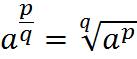for any real numbers (although q may not be zero, since we cannot divide by zero).

Let’s look at the example of 8 to the 2/3 power. First, we raise 8 to the power of the numerator, 2. Then, since the denominator is 3, we take the third root of this number. The expression is read as the cube root of eight squared, and written as: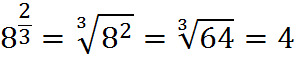When the numerator of the fractional exponent is 1, the expression is a simple root. That is, the 1/2 power is a square root, the 1/3 power is a cubed root, the 1/4 power is a fourth root, etc.

For example, the square root of 9 can be written in exponent format as: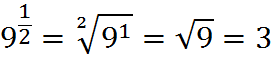Any fractional exponent can also be expressed as a decimal exponent. For example, a square root may also be written as: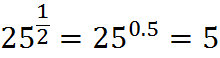Also, decimals which can’t be expressed as a fraction (irrational numbers) may be used as exponents: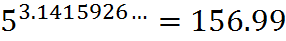The GMAT wouldn’t ask you to make a calculation such as this one exactly, but it might ask you to estimate it. In this case, since 3.1415926 is between 3 and 4, we know that the answer will be between 5 to the third power, which is 125, and 5 to the fourth power, which is 625, considerably closer to 125 than to 625, since 3.1415926 is considerably closer to 3 than to 4.

## Exponents with Fractional or Negative Bases

When a fraction is raised to an exponent, both the numerator and denominator are raised to that exponent: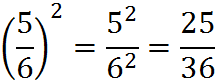Fractions may also be used for both the base and exponent: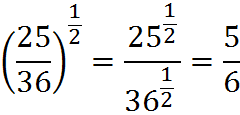In addition, negative fractional exponents may be used, taking the reciprocal of the base, as always, to find the solution: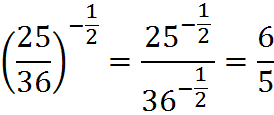As we’ve discussed, negative bases raised to even powers produce positive results, while negative bases raised to odd powers produce negative results.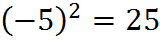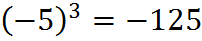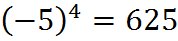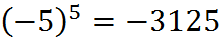Note the difference between these two expressions: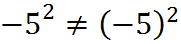The left side is the negative of the square, and the right side is the square of a negative. On the left side, we have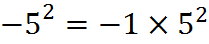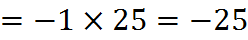and on the right side, we have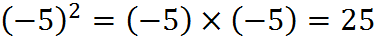They can sound the same when speaking them aloud, so when speaking them aloud, or thinking the words, you can use the word “quantity.” For example: we can describe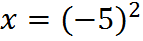unambiguously as “x equals negative 5, quantity squared.”

Roots and fractional/decimal powers are a bit trickier. Odd roots work out fine: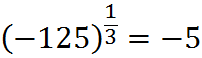Even roots, however, have no real solution: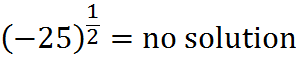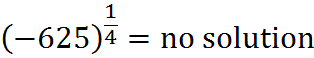## Summary of Exponent Rules

The following rules hold for all integer exponents, provided that the base is non-zero: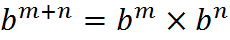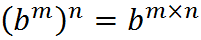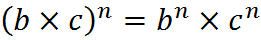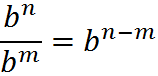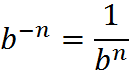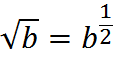Remember all along the way that exponents do nothing more than count the number of times something is multiplied by itself. That fact, along with practice in canceling exponents within fractions, should get you to a point of complete comfort with these rules.

## Comparing Repeated Addition and Repeated Multiplication

Here’s something to chew on: since exponentiation corresponds to repeated multiplication, it has a similarity to multiplication. Multiplication is repeated adding; exponentiation is repeated multiplying. Remembering this can be tremendously useful on GMAT questions. Yes, you need to know and be able to use the exponent rules above, but all along the way, remember that exponents count the number of times something is multiplied.

1. a)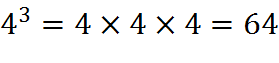b)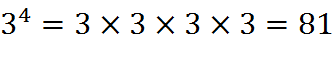c)d)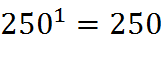2. a)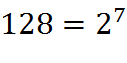b)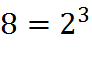c)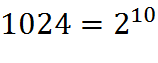3.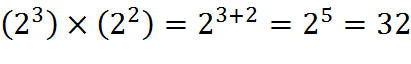4.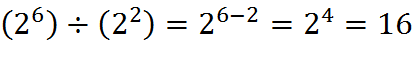5. We can write the following: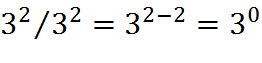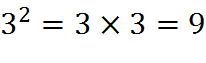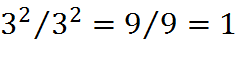From the first and third equations above, we can see that: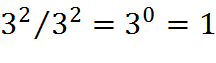The exponent doesn’t have to be 2. The exponent can be any real number and the same logic would work. Any number raised to the 0 power equals 1.

## Table of Whole-Number Exponentials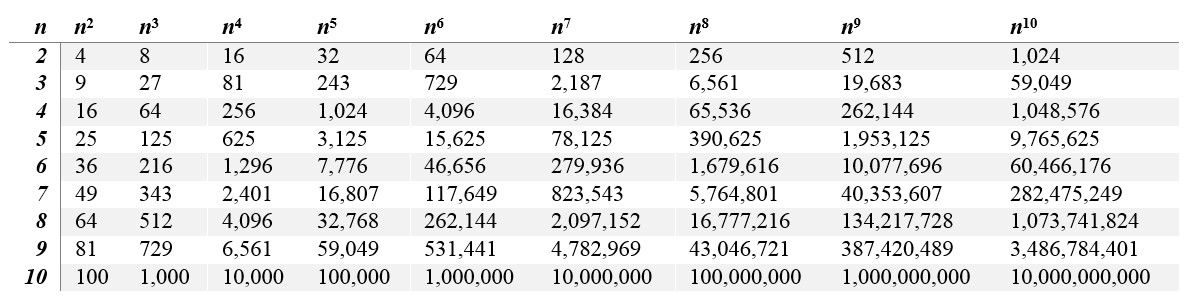## Scientific Notation

Some GMAT questions will present numbers in scientific notation and ask you to work with those numbers. The purpose of scientific notation is to represent extremely small and large numbers, such as these:

123,456,000,000,000,000,000,000,000

0.000 000 000 000 000 000 000 000 000 000 378

With scientific notation, we represent such numbers using exponents. These two numbers, in scientific notation, are: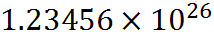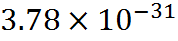Generally, in scientific notation all numbers are written in the form: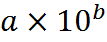where number a has exactly one nonzero digit to the left of the decimal point (a is between -10 and 10). More examples: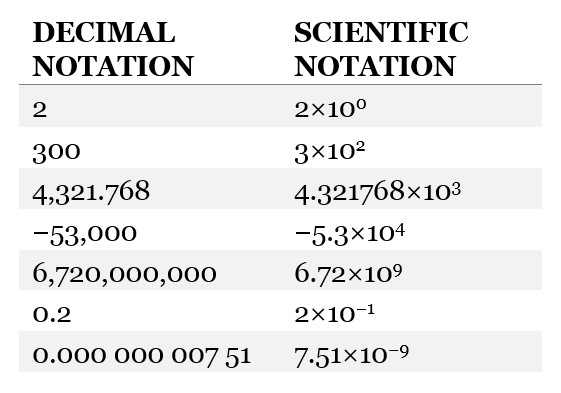## Practice Questions

Powers of Tenth:
http://www.gmatfree.com/powers-of-tenth

Comparing Exponents:
http://www.gmatfree.com/comparing-exponents

Fraction of Decimals and Exponents:
http://www.gmatfree.com/fraction-of-decimals-and-exponents

Welcome! You are encouraged to register with the site and login (for free). When you register, you support the site and your question history is saved.